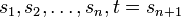# Signed symmetric group of finite degree is a Coxeter group

Suppose$n$ is a nonnegative integer and$G$ is the signed symmetric group of degree$n + 1$. Then,$G$ is isomorphic to a Coxeter group with generators$s_1,s_2,\dots,s_n,t = s_{n+1}$, the$m_{ij}$ are as follows:
•$m_{i(i+1)} = 3$ for$1 \le i \le n - 1$
•$m_{n(n+1)} = 4$
•$m_{ij} = 2$ if$|i - j| > 1$
Under the isomorphism,$s_i$ is idnetified with the transposition$(i,i+1)$ and$t = s_{n+1}$ is the diagonal matrix with the last entry$-1$ and all remaining entries equal to$1$.